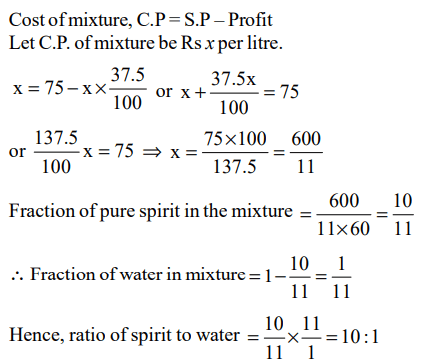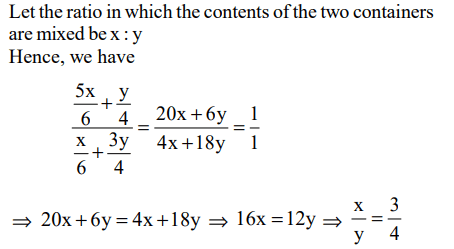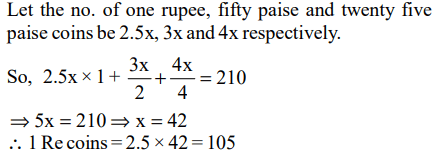## Average, Ratio and Proportion Questions and Answers Part-1

1. A man buys spirit at Rs 60 per litre, adds water to it and then sells it at Rs 75 per litre. What is the ratio of spirit to water if his profit in the deal is 37.5%?
a) 9 : 1
b) 10 : 1
c) 11 : 1
d) None of these

Explanation:2. Two liquids A and B are in the ratio 5 : 1 in container 1 and in container 2, they are in the ratio 1:3, what ratio should the contents of the two containers be mixed so as to obtain a mixture of A and B in the ratio 1:1?
a) 2 : 3
b) 4 : 3
c) 3 : 2
d) 3 : 4

Explanation:3. The average marks of a student in ten papers are 80. If the highest and the lowest scores are not considered, the average is 81. If his highest score is 92, find the lowest
a) 55
b) 60
c) 62
d) Can’t be determined

Explanation: Let the lowest marks be x
Then, ( x + 92 ) + 81 * 8 = 800
x + 92 = 152
x = 60

4. The value of each of a set of silver coins varies as the square of its diameter, if its thickness remains constant, and it varies as the thickness, if the diameter remains constant. If the diameters of two coins are in the ratio 4 : 3, what should the ratio of their thicknesses be if the value of the first is 4 times that of the second?
a) 16 : 9
b) 9 : 4
c) 9 : 16
d) 4 : 9

Explanation:5. I have one rupee coins, fifty paise coins and twenty five paise coins. The number of coins are in the ratio 2.5 : 3 : 4. If the total amount with me is Rs 210. Find the number of one rupee coins.
a) 90
b) 85
c) 100
d) 105

Explanation:6.There are two containers : the first contains 500 ml of alcohol, while the second contains 500 ml of water. Three cups of alcohol from the first container is removed and is mixed well in the second container. Then three cups of this mixture is removed and is mixed in the first container. Let ‘A’ denote the proportion of water in the first container and ‘B’ denote the proportion of alcohol in the second container. Then,
a) A > B
b) A < B
c) A = B
d) Cannot be determined

Explanation: Let capacity of each cup be 100 ml
After first operaton, first container will have 200 ml of alcohol and second container will have 300 l alcohol and 500 ml water.
Ratio of water to alcohol in the second container = 5 : 3.
After second operation, the quantity of water and alcohol left would be $(300\times\frac{5}{8})$  = 187.5 ml and $(300\times\frac{5}{8})$  = 112.5 ml respectively in the first container.
and the quantity of water and alcohol in the first container is 187.5 ml and (200 + 112.5) ml = 312.5 ml
hence, ratio of water and alcohol = 187.5 : 312.5 = 3 : 5
and the ratio of alcohol to water = 5 : 3.
Hence, on comparing ratio of water and alcohol in both the containers we find that A = B

7. Mr Launcher plans to launch a new TV channel called Dekha Dekhi (DD). He envisages a viewership of 42%. He plans to capture the viewership from CAT TV and MAT TV, which currently hold viewership of 35% and 48%, each having a distinct and separate target audience. In what ratio should he capture the target audience of the two channels
a) 6 : 7
b) 7 : 6
c) 1 : 1
d) 8 : 9

Explanation:8. There are seven consecutive natural numbers such that the average of the first five is n. Then average of all seven numbers will be?
a) n
b) n + 1
c) $kn+\frac{1}{k}$ where k is a positive constant
d) $n+\frac{2}{7}$

Explanation: If n is the average of 5 natural numbers. Therefore n should be the middle term.
Then the first five numbers will
n – 2, n – 1, n, n + 1, n + 2
Now, n - 2, n - 1; n, n +1, n + 2, n + 3, n + 4 will be all seven no.
Hence, new average will be (n + 1)

9. Three friends, returning from a movie, stopped to eat at a restaurant. After dinner, they paid their bill and noticed a bowl of mints at the front counter. Sita took 1/3 of the mints, but returned four because she had a momentary pang of guilt. Fatima then took 1/4 of what was left but returned three for similar reasons. Eswari then took half of the remainder but threw two back into the bowl. The bowl had only 17 mints left when the raid was over. How many mints were originally in the bowl?
a) 38
b) 31
c) 41
d) None of these

Explanation:10. Three maths classes: X, Y and Z take an algebra test.
The average score in class X is 83.
The average score in class Y is 76.
The average score in class Z is 85.
The average score of all students in classes X and Y together is 79.
The average score of all students in classes Y and Z together is 81.
What is the average for all the three classes?
a) 81
b) 81.5
c) 82
d) 84.5